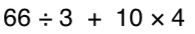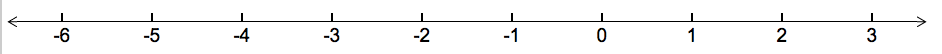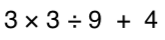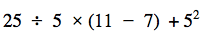Absolute Value
Exponents
Order of Operations
Distributive Property
Fractions, %, and decimals
Rate and Ratio
100

Find the absolute value of:

|-10|100

When you see the following expression, what is the big number called and what is the little number on top of it called?

24

100

Simplify the following expression:100

8(4+2) = ?

48

100

which is largest, 25%, 0.027, or 2/5?

25%

100

Balia knows that 6 cups of rice will feed 15 people.  How many cups of rice are needed to feed 135 people?

54 cups

200

What is greater:

|-6| or |3|The absolute value of a number is the number's distance from zero.

|-6|

200

How can you rewrite 9in expanded form?

9 x 9

200

Simplify the following expression:200

5(x - 3)

5x - 15

200

Which is smallest, .23, 22%, or 1/5?

1/5

200

A train in Japan can travel 813.5 miles in 5 hrs.  Find the unit rate in miles per hour

162.7 mi / hr

300

Place the following in order from smallest number, to biggest number:

|-20|, 18, |12|, |-14|, 8

The absolute value of a number is the number's distance from zero.

8, |12|, |-14|, 18, |-20|

300

How can you rewrite the following in exponential form?

7 x 7 x 7 x 7 x 7

75

300

3 + 42 * (3 + 2)

83

300

7 + 9(2x - 3)

18x - 20

300

what is 236% written as a decimal?

2.36

300

Arrange in fasted to slowest:

Abel reads 50 pages in 45 minutes; Brian reads 90 pages in 75 minutes; Charlie reads 175 pages in 2 hours

Charlie, Brian, Abel

400

What is:

|-7| + |-2|

The absolute value of a number is the number's distance from zero.

9

40045

400

-3(-x - 10)

3x + 30

400

what is 4/5 written as a percent?

80%

400

An ice skater covered 1500 meters in 106 seconds.  What is her unit rate in meters per second

14.15 m per second

500

2 x |-5| + |4| - 14

Remember to use your order of operations.

0

500

What is the value of:

23 + 32 - 42

50012

500

6 + (-2 + -5)

-1

500

x + 10 - 6(x - 3x) + 4

13x + 14

500
What is 0.34 written as a fraction?

34/100 or 17/50

500

Arrange from most expensive to least expensive if they buy lunch 5 days per week:

Alice spends $3 per day; Betty spends$25 every 2 weeks; Cindy spends \$75 per month (assume 4 weeks in a month)

Cindy, Alice, Betty

Click to zoom